Détails de la société

• GUANGZHOU VICPAS TOUCH TECHNOLOGY CO.,LTD

•  [Guangdong,China]
• Type d'Affaires:Fabricant , Société de commerce
• Main Mark: À l'échelle mondiale , Afrique , Amériques , Asie , Caraïbes , Europe de l'Est , L'Europe  , moyen-Orient , Europe du Nord , Océanie , Autres marchés , Europe occidentale
• Exportateur:51% - 60%
• certs:CE
Panier (0)

GUANGZHOU VICPAS TOUCH TECHNOLOGY CO.,LTD

Groupes de Produits
•Écran LCD HMI
service en ligne
Informations sur l'industrie

# The structure of the resistive touch screen glass and the principle of the touch panel

2019-01-17

Many LCD modules use resistive touch screens. These touch screens are equivalent to sensors that convert physical locations to voltage values that represent X and Y coordinates. There are usually 4-wire, 5-wire, 7-wire and 8-wire touch screens. This article describes in detail the structure of the SAR, the structure and implementation of the four touch screens, and the methods for detecting touch. The structure of the resistive touch screen and the principle of the touch screen
Many LCD modules use resistive touch screens. These touch screens are equivalent to sensors that convert physical locations into voltage values representing X and Y coordinates. There are usually 4-wire, 5-wire, 7-wire and 8-wire touch screens. This article details the structure of SAR, the structure and implementation of four touch screens, and the method of detecting touch.

A resistive touch screen is a sensor that converts the physical position of a touch point (X, Y) in a rectangular area into a voltage representing X and Y coordinates. Many LCD modules use resistive touch screens. This screen can use four, five, seven or eight lines to generate the screen bias voltage and read back the voltage at the touch point.

In the past, in order to read the touch point coordinates on a resistive touch screen into a microcontroller, a dedicated touch screen controller chip was used, or a microcontroller's on-chip analog-to-digital converter (ADC) was connected using a complex external switch network. Sharp's LH75400 / 01/10/11 series and LH7A404 microcontrollers feature an on-chip ADC with a touch screen bias circuit that uses a successive approximation register (SAR) type converter. Using these controllers, the direct interface between the touch screen sensor and the microcontroller controls all touch screen bias voltages without CPU intervention and records all measurements. This article will detail the structure and implementation of four-wire, five-wire, seven-wire and eight-wire touch screens.

Figure 6 Touch Screen Resistance Calculation Method

There are many ways to implement SAR SAR, but its basic structure is very simple. See Figure 1.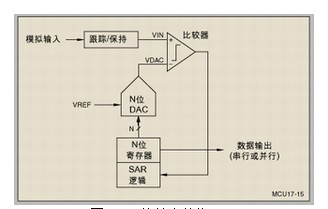Figure 1. Basic structure of the SAR. This structure stores the analog input voltage (VIN) in sample/hold and sets the N-bit register to the middle value (ie, 100 ..... 0, where the highest bit is set to 1). Perform a binary lookup algorithm. Therefore, the output of a digital-to-analog converter (DAC) (VDAC) is half of VREF, where VREF is the reference voltage of the ADC. After that, the comparison operation is performed again to determine if VIN is less than or greater than VDAC:

1. If VIN is less than VDAC, the comparator output logic is low and the most significant bit of the N-bit register is cleared.

2. If VIN is greater than VDAC, the comparator outputs a logic high (or 1) and the most significant bit of the N-bit register remains at 1.

Thereafter, the control logic of the SAR

moves to the next bit, forcing the bit to be high, and then performing the next comparison. The SAR control logic will repeat the above sequence of operations until the last bit. After the conversion is complete, there is an N-bit data word in the register.

Figure 2 shows an example of a 4-bit conversion process where the Y-axis and the thick line represent the output voltage of the DAC.

Fig. 1 The basic structure of the SAR This structure stores the analog input voltage (VIN) in a track/hold, and the N-bit register is set to the middle value (ie, 100.....0, where the highest bit is set to 1). Perform a binary lookup algorithm. Therefore, the output of the digital-to-analog converter (DAC) (VDAC) is one-half of VREF, where VREF is the reference voltage of the ADC. After that, perform a comparison operation again to determine if VIN is less than or greater than VDAC:

1. If VIN is less than VDAC, the comparator output logic is low and the highest bit of the N-bit register is cleared.

2. If VIN is greater than VDAC, the comparator output logic high (or 1) and the highest bit of the N-bit register remains at 1.

Thereafter, the control logic of the SAR moves to the next bit, forcing the bit to be high, and then performing the next comparison. The SAR control logic will repeat the above sequence of operations until the last bit. When the conversion is complete, an N-bit data word is available in the register.

Figure 2 shows an example of a 4-bit conversion process where the Y-axis and thick line represent the output voltage of the DAC.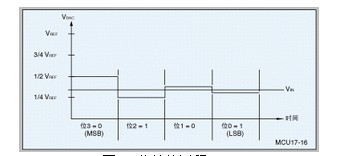Figure 2 shows the 4-bit conversion process in this example:

1. The first comparison shows that VIN is less than VDAC, so bit  is set to zero. Then set the DAC to 0b0100 and perform a second comparison.

2. In the second comparison, VIN is greater than VDAC, so bit  remains at 1. Subsequently, the DAC is set to 0b0110 and a third comparison is performed.

3. In the third comparison, Bit is set to 0. Then the DAC is set to 0b0101 and the last comparison is performed.

4. In the last comparison, Bit  remains at 1 because VIN is greater than VDAC.

Touch screen principle The touch screen consists of two transparent layers stacked on each other. The four- and eight-wire touch screens consist of two layers of transparent resistive material with the same surface resistance. The five-wire and seven-wire touch screens consist of a resistive layer and a conductive layer. It is also common to use elastic materials to separate the two layers. Contact between the top layer and the bottom layer occurs when the pressure on the surface of the touch screen (eg, with a pen tip or finger) is sufficiently large. All resistive touch screens use the voltage divider principle to generate voltages that represent the X and Y coordinates. As shown in Figure 3, the voltage divider is implemented by connecting two resistors in series. The upper resistor (R1) is connected to the positive reference voltage (VREF) and the lower resistor (R2) is grounded. The voltage measurement of the two resistor junctions is proportional to the resistance of the next resistor.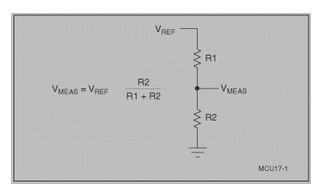Figure 3. A voltage divider in series with two resistors measures the coordinates of a particular direction on a resistive touch screen. The resistor layer needs to be biased: one side is connected to VREF and the other side is connected to ground. At the same time, connect the unbiased layer to the high impedance input of the ADC. When the pressure on the touch screen is large enough to make contact between the two layers, the resistive surface is divided into two resistors. Their resistance is proportional to the distance from the touch point to the offset edge. The resistance between the contact and ground corresponds to the lower resistance in the voltage divider. Therefore, the voltage measured on the unbiased layer is proportional to the distance from the touch point to the ground.

The four-wire touch screen four-wire touch screen contains two resistive layers. One has a vertical bus on the left and right edges of the screen, and the other has a horizontal bus at the bottom and top of the screen. See Figure 4. To measure in the X-axis direction, the left bus bias is 0V and the right bus bias is VREF. Connect the top or bottom bus to the ADC and measure when the top and bottom layers are in contact.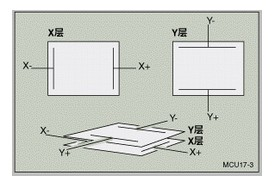The two resistive layers of the four-wire touch screen of Figure 4 are measured in the Y-axis direction with the top bus biased at VREF and the bottom bus biased at 0V. The ADC input is connected to the left or right bus and the voltage can be measured when the top and bottom layers are in contact. Figure 5 shows a simplified model of a four-wire touch screen with two layers of contact. For a four-wire touch screen, the ideal connection method is to connect the bus and offset VREF to the positive reference input of the ADC and set the bus to 0V to the negative reference input of the ADC.

The five-wire touch screen five-wire touch screen uses a resistive layer and a conductive layer. The conductive layer typically has contacts on one side of its edge. Each of the four corners of the resistive layer has one contact. To make measurements in the X-axis direction, offset the upper left and lower left corners to VREF and ground in the upper right and lower right corners. Since the left and right corners have the same voltage, the effect is similar to the effect of connecting the left and right buses, similar to the method used in the four-wire touch screen.

In order to measure in the Y-axis direction, the upper left and upper right corners are offset to VREF, and the lower left and lower right corners are offset to 0V. Since the upper and lower corners are the same voltage, the effect is similar to the effect of the bus connecting the top and bottom edges, similar to the method used in a four-wire touch screen. The advantage of this measurement algorithm is that it keeps the voltages in the upper left and lower right corners unchanged; however, if grid coordinates are used, the X and Y axes need to be inverted. For a five-wire touch screen, the best way to connect is to connect the upper left corner (offset to VREF) to the positive reference input of the ADC and the lower left corner (offset to 0V) to the negative reference input of the ADC.

The seven-line touch screen seven-line touch screen is implemented in the same way as the five-line touch screen, except that it adds a line to the upper left and lower right corners. When performing a screen measurement, connect one line in the upper left corner to VREF and the other line to the positive reference line of the SAR ADC. At the same time, one line in the lower right corner is connected to 0V and the other line is connected to the negative reference of the SAR ADC. The conductive layer is still used to measure the voltage across the voltage divider.

In addition to the eight-line touch screen that adds lines to each bus, the eight-wire touch screen is implemented in the same way as a four-wire touch screen. For the VREF bus, one line is used to connect VREF and the other line is the positive reference input of the DAC ADC's digital-to-analog converter. For the 0V bus, one line is used to connect 0V and the other line is used as the negative reference input for the digital-to-analog converter of the SAR ADC. Any of the four lines on the unbiased layer can be used to measure the voltage across the voltage divider.

Detecting Touch All touch screens can be detected by pulling up one of the layers using a weak pull-up resistor and pulling down another layer using a strong pull-down resistor. If the measured voltage of the pullup layer is greater than a certain logic threshold, there is no touch, and if not, there is a touch. The problem with this approach is that the touch screen is a huge capacitor and in addition it may be necessary to increase the capacitance of the touch screen leads to filter out the noise introduced by the LCD. A weak pull-up resistor connected to a large capacitor increases the rise time and may result in the detection of a false touch.

The four-wire and eight-wire touch screens can measure the contact resistance RTOUCH.RTOUCH in Figure 5 to be roughly proportional to the touch pressure. To measure touch pressure, you need to know the resistance of one or two layers in the touch screen. The formula in Figure 6 gives the calculation method. It should be noted that if the measured value of Z1 is close to or equal to 0 (when the contact is close to the grounded X bus during the measurement), some problems will occur in the calculation, and this problem can be effectively improved by using the weak pull-up method.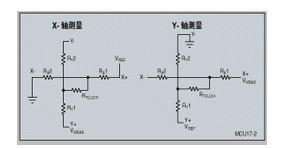Figure 5 RTOUCH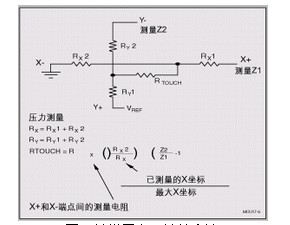Figure 6 Touch Screen Resistance Calculation Method

Related Industry Information
Contacter le Fournisseur?fournisseurMr. Kandy
Que puis-je faire pour vous?